# Nitrogen (two or more N atoms)

Quick move to entries starting with the letters Az, C, D, F, H, I, K, N, O, Q, S, T, U or V.

aldazines:

Azines of aldehydes: RCH=NN=CHR.

Compounds having the structure RC(NH2)=NOH and derivatives formed by substitution; formally the oximes of carboxamides. NOC Rule C-952.

Derivatives of oxoacids RnE(=O)OH in which the hydroxy group is replaced by an amino group and the oxo group is replaced by =NR. Amidines include carboxamidines, sulfinamidines and phosphinamidines, R2P(=NR)NR2. In organic chemistry an unspecified amidine is commonly a carboxamidine. See also carboxamidines, sulfinamidines.

Cations formally derived by the addition of one hydron to the N or O atom of an amide and N-hydrocarbyl derivatives thereof. In organic chemistry an unspecified amidium ion is commonly a carboxamidium ion: RC(OH)=N+R2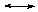RC(=O+H)NR2 or RC(=O)N+R3. The term does not imply knowledge concerning the position of the cationic centre. RNRI Rule RC-82.1.2. E.g. PhC(=O)N+Me 3 N,N,N-trimethylbenzamidium.

Compounds having the structure RC(=NH)NHNH2 or RC(NH 2)=NNH2, formally derived from carboxylic acids. These tautomers are named hydrazide imides and amide hydrazones, respectively. NOC Rule C-953. Also included are N-hydrocarbyl derivatives.

Compounds having two amino groups bonded to the same carbon, R2C(NR2)2. Also called geminal diamines. [The term aminal has also been used, with consequent ambiguity, for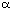-amino ethers (hemiaminal ethers); such use is discouraged.] Cf. hemiaminals.

Compounds formally derived from the attachment of an amine R3N to a nitrene RN:. The structure R3N+-N--R expresses the 1,2-dipolar character of amine imides. They may be named systematically as substituted diazan-2-ium-1-ides. RNRI Rule RC-84.1.1. E.g. Me3N+-N-Me 1,2,2,2-tetramethyldiazan-2-ium-1-ide or trimethylamine N-methylimide. See also ylides.

amine imines: An undesirable synonym for amine imides. See under imides (2), ylides.

aminonitrenes: An incorrect name for isodiazenes. See under carbene analogues.

An undesirable synonym for amine imides. See under ylides.

Saturated acyclic nitrogen hydrides having the general formula NnHn+2.

1. Compounds bearing the group -N3, viz. -N=N+=N-; usually attached to carbon. NOC Rule C-941.1. E.g. PhN3 phenyl azide or azidobenzene.

2. Salts of hydrazoic acid, HN3. E.g. NaN3 sodium azide.

A commonly used but undesirable term for azo imides. (Should not be confused with 'azimino', the name for the bridging group, -N=NNH- . NOC Rule B-15.1.)

Condensation products, R2C=NN=CR2, of two moles of a carbonyl compound with one mole of hydrazine . NOC Rule C-923.1. See also aldazines and ketazines. (This term should not be confused with the ending -azine appearing in Hantzsch-Widman names for some heterocycles.)

Derivatives of diazene (diimide), HN=NH, wherein both hydrogens are substituted by hydrocarbyl groups. NOC Rules C-911, C-912. GNOC Recom. R-5.3.3.1 E.g. PhN=NPh azobenzene or diphenyldiazene.

N-Imides of azo compounds, analogous to azoxy compounds, having a delocalised structure RN=N+(R)N-RRN-N+(R)=NR; commonly but undesirably referred to as azimines. See under dipolar compounds, imides (2), ylides.

The 1,3-dipolar N-imides of azomethines having the structure RN--N+(R)=CR2RN=N+(R)C-R2. The term azo ylides, derived from the second resonance form, has also been used. See under imides (2), ylides.

N-Oxides of azo compounds, of structure RN=N+ (O-)R. NOC Rule C-913. GNOC Recom. R-5.3.3.2. See under dipolar compounds. E.g. PhN=N+(O-)Ph azoxybenzene or diphenyldiazene oxide.

Carbodiimide, HN=C=NH, and its hydrocarbyl derivatives. NOC Rule C-956.

Compounds having the structure RC(=NR)NR2. The term is used as a suffix in systematic nomenclature to denote the -C(=NH)NH2 group including its carbon atom. NOC Rule C-951.1. E.g. CH3C(=NH)NH2 acetamidine,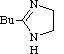2-butyl-4,5-dihydroimidazole.

Synthetic dyes with the general formula R2N[CH=CH]nCH=N+R2R2N+=CH[CH=CH]nNR2 (n is a small number) in which the nitrogen and part of the conjugated chain usually form part of a heterocyclic system, such as imidazole, pyridine, pyrrole, quinoline and thiazole. E.g.

F. M. Hamer, The Cyanine Dyes and Related Compounds, Interscience, 1964.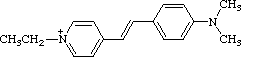diamidides:

Analogues of acyclic carboxylic acid anhydride in which =O has been replaced by =NR and -O- by -NR-: RC(=NR)NRC(=NR)R, N-imidoyl amidines. The name 1,3,5-triazapentadienes, formed in contravention of NOC Rule C-61, is sometimes applied as a class name to diamidides. See imidines .

diazanylidenes: An alternative term for isodiazenes.

An incorrectly formed name for isodiazenes. Use of this name is discouraged. See also nitrenes.

Compounds having the divalent diazo group, =N+=N-, attached to a carbon atom. See also dipolar compounds. E.g. CH2=N2 diazomethane.

Compounds having the structure RN=N-NR2 (not all R = H, and one R commonly aryl). See also triazenes. In systematic nomenclature, diazoamino prefixed to the name of RH names the compound RN=NNHR' (R = R'). NOC Rule C-942.2. E.g. PhN=N-NPhMe N-methyldiazoaminobenzene.

Salts RN=NO- M+ (R commonly aryl) of the compounds RN=NOH, (hydrocarbon)diazohydroxides. NOC Rule C-931.3. E.g. PhN=NO- K+ potassium benzenediazoate. In GNOC Recom. R-5.3.3.4 these compounds are named as hydrocarbyldiazenolates and hydrocarbyldiazenols respectively. E.g. PhN=NOH phenyldiazenol. RN=NO- M+ are also known by the non-IUPAC name diazotates.

Compounds of structure RN2+ Y-, in which R is generally but not necessarily aryl, and the cations of which are usually formulated as RN+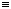N. NOC Rule C-931. E.g. PhN+N Cl- benzenediazonium chloride. They may also be named, from the canonical form RN=N+, hydrocarbyldiazenylium salts. RNRI Rule RC-82.2.2.3.

Diazocyclohexadienones, which may also be considered as dipolar diazonio phenoxides, obtained by diazotizing aromatic primary amines having a hydroxy group in an ortho or para position. Also known as diazophenols. See also diazo compounds, phenoxides , quinone diazides.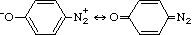diazotates: See diazoates.

The compound H2NC(=NH)SSC(=NH)NH2 and its derivatives formed by substitution at nitrogen. NOC Rule C-951.5.

The parent compound H2NN=CHN=NH and its derivatives formed by substitution at carbon and/or nitrogen. NOC Rule C-955.1.

Compounds derived from oxoacids RkE(=O)l(OH)m (l not equal to 0) by replacing -OH by -NRNR2 (R groups are commonly H), as in carbohydrazides, RC(=O)NHNH2, NOC Rule C-921.5, (GNOC Recom. R-5.7.8.4), sulfonohydrazides, RS(=O)2NHNH2, (NOC Rule C-641.9); and phosphonic dihydrazides, RP(=O)(NHNH2)2.

Compounds, RC(-NHNH2)=NNH2, derived from carboxylic acids by replacing -OH by -NHNH2 (or N-substituted analogues) and =O by =NNH2 (or substituted analogues). NOC Rule C-954.1. A specific hydrazidine is named as a hydrazide hydrazone. E.g. hexanohydrazide hydrazone.

Hydrazine (diazane), H2NNH2, and its hydrocarbyl derivatives. When one or more substituents are acyl groups, the compound is a hydrazide. NOC Rule C-921. N-Alkylidene derivatives are hydrazones. Cf. azines, hydrazo compounds.

hydrazinylidenes: Synonymous with isodiazenes. RNRI Rule RC-81.1.3.2.

Compounds containing the divalent hydrazo group, -NHNH-, such as hydrazoarenes (1,2-diarylhydrazines or 1,2-diaryldiazanes, usually with both aryl groups the same) and their N-substituted derivatives: ArNRNRAr. See also hydrazines. NOC Rule C-921.2.

Compounds having the structure R2C=NNR2, formally derived from aldehydes or ketones by replacing =O by =NNH2 (or substituted analogues). NOC Rule C-922.

Compounds derived from oxoacids RkE(=O)l(OH)m (l not equal to 0) by replacing a double-bonded oxygen atom by =NNR2 , as in carbohydrazonic acids, RC(OH)=NNH2 (NOC Rule C-451.1) and sulfonohydrazonic acids, RS(=O)(=NNH2)OH (GNOC Recom. R-5.7.2.1).

Nitrenes having the structure RC(=NR)N:RC(-N-R)=N+:. See imidoyl carbenes.

Compounds having the structure R2NN:.R2N+=N-. GNOC Recom. R-5.3.5. These compounds can also be called diazanylidenes or hydrazinylidenes. RNRI Rule RC-81.1.3.2. They have also been called by the trivial name azamines and the incorrectly formed name 1,1-diazenes. They should not be called aminonitrenes; see under carbenes.

ketazines:

Azines of ketones: R 2C=NN=CR2.

Amines substituted at N with a nitro group (a contracted form of N-nitroamines); they are thus amides of nitric acid, and the class is composed of nitramide, O2NNH2, and its derivatives formed by substitution.

Ylides having the structure RCN+-N--RRC+=NN--RRC-=N+=NR. Also known as nitrile imines or nitrilimines, especially in the German literature. R. Huisgen, in 1,3-Dipolar Cycloaddition Chemistry, A. Padwa, Ed. John Wiley and Sons, 1984, p. 3. See also dipolar compounds, ylides.

Compounds having the structure O2NN=CR2 (also called N-nitroimines).

Compounds having the structure RC(=NOH)NO2 .

Although this term has, regrettably, been used to mean the N-nitroso derivatives of amides, logically it refers to amides of nitrous acid; the unstable parent compound H2NNO is, in fact, named nitrosamide, but its derivatives formed by substitution with hydrocarbyl groups are by long custom called nitrosamines. See also nitramines.

N-Nitroso amines: compounds of the structure R2NNO. Compounds RNHNO are not ordinarily isolable, but they, too, are nitrosamines. The name is a contraction of N-nitrosoamine and, as such, does not require the N locant. See also nitrosamides.

N-Nitroso imines: O=NN=CR2.

Hypothetical compounds having the structure RC(NH2)3, and N-substituted derivatives thereof. (R2N)4C are generally included, but such use is obsolescent.

A potentially confusing term for diazooxides; the presence of an azido group, -N3, is falsely suggested.

Compounds having the structure R2C=NNHC(=O)NH2, formally derived by condensation of aldehydes or ketones with semicarbazide [NH2NHC(=O)NH2]. NOC Rule C-982.

Compounds having the structure R2C=NNHC(=O)C(=O)NH2, formally derived from the condensation of aldehydes or ketones with semioxamazide (the hydrazide of oxamic acid), H2NNHC(=O)C(=O)NH2.

Triazane, NH2NHNH2, and its hydrocarbyl derivatives. NOC Rule C-942.

Triazene, NH2N=NH, and its hydrocarbyl derivatives. See also diazoamino compounds. NOC Rule C-942.1.

Salts of O-hydronated urea, having the structure [HOC(=NH2)NH2]+ X- (and O- and N-hydrocarbyl derivatives). NOC Rule C-973.1.

Relatively stable delocalized radicals, derived from hydrazyl, H2NN.H, of the types shown, in particular the six-membered ring: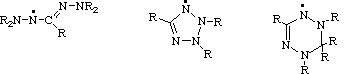(R most commonly aryl groups.)